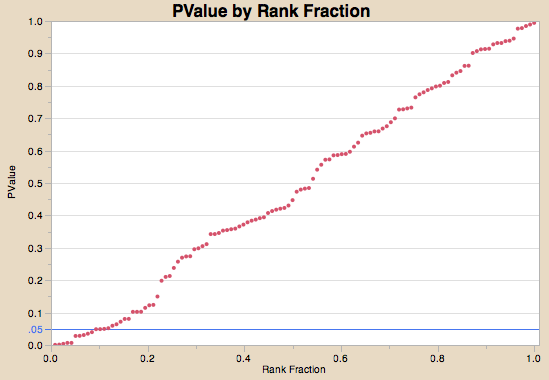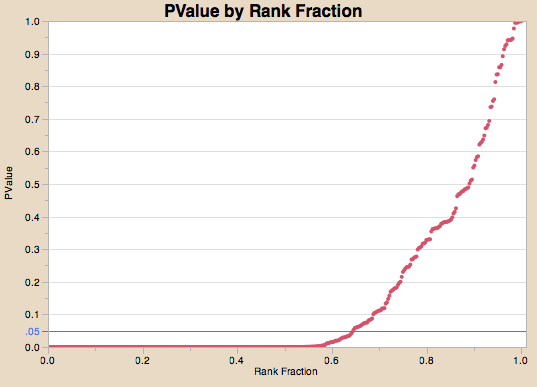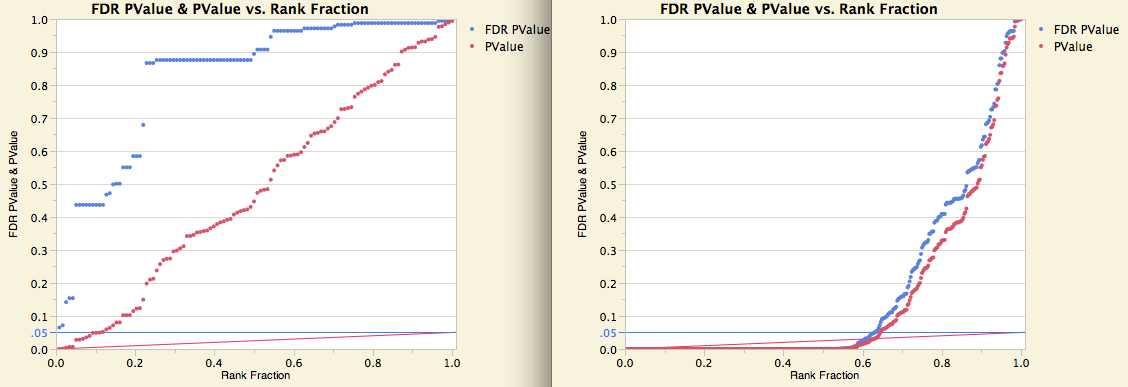Our World Statistics Day conversations have been a great reminder of how much statistics can inform our lives. Do you have an example of how statistics has made a difference in your life? Share your story with the Community!
Choose Language Hide Translation Bar
Not just filtering coincidences: False discovery rate

Purely random data has a 5% chance of being significant. Choose the most significant p-values from many tests of random data, and you will filter out the tests that are significant by chance alone.

Suppose we have a process that we know is stable and consistent. We measure lots of responses, and we also have a Lot ID. The question is: Does any response differ significantly across lots? So we run ANOVAs on them, extract the p-values, sort them, and see this, from the smallest to the largest. We have 13 tests that are significant, i.e., below the line at .05. With random data, we expect around 5% to be significant, which is .05*118 = 5.9, so we have a few more than we would expect.Notice the distribution of p-values — across the fraction of the data, they follow a straight line from near 0 to 1. This is the characteristic of a uniform distribution of values. For purely random independent tests, p-values follow a uniform distribution. We pretty much know that there are no real differences here, yet 13 tests are significant. Our main goal is to look for big differences, so we always want to sort and filter by p-values. But we need something to adjust for looking at lots of tests. Otherwise, we have selection bias — multiple-test bias — a coincidence filter.

Now that we have seen the pattern from data where there are no differences, let’s see what it can look like when many of the tests are really significant. Here, more than 60% of the data looks significant.Looking at the right side the graph, it looks like all the values above .05 follow somewhat of a straight line toward 1, but around .10 or .05, the slope of the line seems to change a lot, and it gets very dense near the bottom. Looking at p-values like this – looking for a change in slope – turns out to be the subject of a paper by Schweder and Spjotvoll (Biometrika 1982, p493-502) that started researchers thinking about looking at the whole population of p-values. The reasoning was similar to an analogous situation that Cuthbert Daniel noticed more than 20 years earlier when you plotted estimates from a factorial experiment – the small values near the middle seemed to follow a normal quantile line, but the significant effects veered off that slope.

Well, it happens that there is a vast literature on multiple test bias and methods of adjusting the tests to account for them. These techniques worked well when there were only a few means to compare or tests to make. One very simple procedure was to just ask that the p-values be less than alpha/m where m is the number of tests, and alpha is the significance level. In 1961, O.J. Dunn proved (using the Bonferroni inequality) that this criterion gave you overall protection for all the tests.

If we look at the first set of p-values, the lowest p-value is 0.00055, and there are 118 tests. So our criterion is .05/118= 0.0004, and we conclude that there is nothing significant.

But the Bonferroni is a very conservative criterion, making it difficult for most experiments involving many tests to obtain results, so many other methods were developed.

In 1995, Benjamini and Hochberg (BH) changed the game completely. Instead of controlling for whether any differences were falsely declared significant, they changed the criteria to controlling for the rate at which declarations of significance were false. If your tolerance for false discovery was 5%, then you just had to make sure the expected number of false discoveries was only 5% of the ones you declared significant. They were able to construct a very simple method to control for the false discovery rate. You sort your p-values from high to low. For the highest p-value, you can use the false discovery rate (FDR) rate alpha, e.g., .05 as the criterion. For each next highest p-value, your criterion shrinks a little, subtracting alpha/m, forming a ramp. At the bottom of the ramp is the criterion for the smallest p-value, which is alpha/m, the Bonferroni criterion. Any p-values that fall under that ramp are declared significant, and BH proved that for independent tests, you control for the false discovery rate with this method.On the left is the lot-to-lot data, and the Benjamini-Hochberg ramp is shown by the red line, starting at .05 on the right, down to .05/118, and none of the red p-values manages to get under the ramp. On the right is the engineering change data; the red points dive under the ramp such that more than 60% of the responses are significantly different.

You can also transform the p-value such that the new FDR-adjusted p-value has the false discovery property when compared with the false discovery rate, e.g., .05. The FDR-adjusted p-values are shown by the blue markers, which dive under the blue line at .05 for the same test that the red marker dive under the red line. Notice that when the p-values seem uniformly distributed in the left side, the FDR-adjusted p-values are adjusted upward a lot. But when the p-values head down rapidly going from right to left, the FDR-adjustment is much less.

What if the tests are not independent? In most cases, specifically when there is a positive correlation between the tests, it turns out that the BH FDR adjustment is conservative, giving you the protection you want. The false discovery rate idea has since grown a rich literature, including refinements worth doing in some cases.

In the era of Big Statistics when you conduct hundreds, thousands or even millions of tests, the FDR-adjusted p-values should be the new standard best practice. You won’t just be filtering out coincidences; you will know that most of what you catch will be real effects.

You can get the False Discovery Rate PValue add-in from the JMP File Exchange. (Download requires free SAS profile.) Also FDR adjustments are standard in the Response Screening platform and fitting personality in JMP 11.

Note: This is part of a Big Statistics series of blog posts by John Sall. Read all of his Big Statistics posts.

Article Labels

There are no labels assigned to this post.

Article Tags